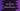# C program to pass a two-dimensional array to a different function# Two-dimensional array in C :

In this c programming tutorial, we will learn how to pass one two dimensional arrays to a different function and print out the content of the array. The program will take the inputs of the array from the user and populate the array. It will pass the array to a different function and print out the contents.

## Algorithm :

1. Ask the user to enter the row and column count of the array.
2. Read the values and store in different variables.
3. Using two loops, take the inputs and populate the array.
4. Pass the array to a different function.
5. Using two for loops, print out the content of the array in the function.

### C program :

``````#include<stdio.h>

//7
void print(int m, int n, int arr[m][n])
{
//8
int i, j;
//9
for (i = 0; i < m; i++)
{
for (j = 0; j < n; j++)
{
printf("%d ", arr[i][j]);
}
//10
printf("\n");
}
printf("\n");
}

int main()
{
//1
int rowCount, columnCount;
int i, j;

//2
printf("Enter the row count : ");
scanf("%d", &rowCount);

//3
printf("Enter the column count : ");
scanf("%d", &columnCount);

//4
int array[rowCount][columnCount];

//5
for (i = 0; i < rowCount; i++)
{
for (j = 0; j < columnCount; j++)
{
printf("Enter value for array[%d][%d] : ", i, j);
scanf("%d", &array[i][j]);
}
}

//6
print(rowCount, columnCount, array);
return 0;
}``````

### Explanation :

The commented numbers in the above program denote the step numbers below :

1. Create integer variables rowCount and columnCount to hold the row and column count of the array. Integers_ i_ and j to use in the loop.
2. Ask the user to enter the row count. Read it and store it in the rowCount variable.
3. Similarly, ask the user to enter the column count. Read it and store it in the columnCount variable.
4. Create one two dimensional array by using the user provided row and column count.
5. Using two for loops, read and populate the array by taking the inputs from the user.
6. Call the print method to print the content of the array.
7. print method takes three parameters: the first one is the row count, the second one is the column count and the third one is the array.
8. Create two integer variables i and_ j_.
9. Iterate through the array by using two for loops. Print all rows of the array one by one.
10. Add one new line after printing a row.

### Sample Output :

``````Enter the row count : 3
Enter the column count : 3
Enter value for array : 1
Enter value for array : 2
Enter value for array : 3
Enter value for array : 4
Enter value for array : 5
Enter value for array : 6
Enter value for array : 7
Enter value for array : 8
Enter value for array : 9
1 2 3
4 5 6
7 8 9

Enter the row count : 3
Enter the column count : 4
Enter value for array : 1
Enter value for array : 3
Enter value for array : 5
Enter value for array : 7
Enter value for array : 9
Enter value for array : 10
Enter value for array : 11
Enter value for array : 23
Enter value for array : 45
Enter value for array : 66
Enter value for array : 77
Enter value for array : 54
1 3 5 7
9 10 11 23
45 66 77 54``````

### Conclusion :

We have learned how to pass a two-dimensional array to a different function and how to print the content of the array. Instead of using a for loop, we can also use one while loop to solve the same problem. Try to run the example above and drop one comment if you have any queries.